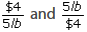Definition Of Rate

Rate is a ratio that compares two quantities of different units.

Unit Rate: Unit rate is a rate in which the second term is 1.
For example, Jake types 10 words in 5 seconds.
Jake's unit rate is the number of words he can type in a second.
His unit rate is 2 words per second.

Examples of Rate

20 oz of juice for $4, miles per hour, cost per pound etc. are examples of rate. Video Examples: Rate of Change Solved Example on Rate Ques:"4 lb of meat costs$5." Identify the two rates given by the statement.

Choices:

A.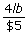and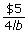B.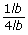andC.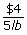and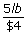D. none of these

Step 2: The two rates given by the statement are